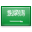# Disc integration

Disc integration, also known in integral calculus as the disc method, is a means of calculating the volume of a solid of revolution of a solid-state material when integrating along an axis "parallel" to the axis of revolution. This method models the resulting three-dimensional shape as a stack of an infinite number of discs of varying radius and infinitesimal thickness. It is also possible to use the same principles with rings instead of discs (the "washer method") to obtain hollow solids of revolutions. This is in contrast to shell integration which integrates along an axis perpendicular to the axis of revolution.

## Definition

### Function of x

If the function to be revolved is a function of x, the following integral represents the volume of the solid of revolution:

${displaystyle pi int _{a}^{b}R(x)^{2},dx}$where R(x) is the distance between the function and the axis of rotation. This works only if the axis of rotation is horizontal (example: y = 3 or some other constant).

### Function of y

If the function to be revolved is a function of y, the following integral will obtain the volume of the solid of revolution:

${displaystyle pi int _{c}^{d}R(y)^{2},dy}$where R(y) is the distance between the function and the axis of rotation. This works only if the axis of rotation is vertical (example: x = 4or some other constant).

### Washer method

To obtain a hollow solid of revolution (the “washer method”), the procedure would be to take the volume of the inner solid of revolution and subtract it from the volume of the outer solid of revolution. This can be calculated in a single integral similar to the following:

${displaystyle pi int _{a}^{b}left(R_{mathrm {O} }(x)^{2}-R_{mathrm {I} }(x)^{2} ight),dx}$where RO(x) is the function that is farthest from the axis of rotation and RI(x) is the function that is closest to the axis of rotation. One should take caution not to evaluate the square of the difference of the two functions, but to evaluate the difference of the squares of the two functions.

${displaystyle R_{mathrm {O} }(x)^{2}-R_{mathrm {I} }(x)^{2} ot equiv left(R_{mathrm {O} }(x)-R_{mathrm {I} }(x) ight)^{2}}$(This formula only works for revolutions about the x-axis.)

To rotate about any horizontal axis, simply subtract from that axis each formula. If h is the value of a horizontal axis, then the volume equals

${displaystyle pi int _{a}^{b}left(left(h-R_{mathrm {O} }(x) ight)^{2}-left(h-R_{mathrm {I} }(x) ight)^{2} ight),dx,.}$For example, to rotate the region between y = −2x + x2 and y = x along the axis y = 4, one would integrate as follows:

${displaystyle pi int _{0}^{3}left(left(4-left(-2x+x^{2} ight) ight)^{2}-(4-x)^{2} ight),dx,.}$The bounds of integration are the zeros of the first equation minus the second. Note that when integrating along an axis other than the x, the graph of the function that is farthest from the axis of rotation may not be that obvious. In the previous example, even though the graph of y = x is, with respect to the x-axis, further up than the graph of y = −2x + x2, with respect to the axis of rotation the function y = x is the inner function: its graph is closer to y = 4 or the equation of the axis of rotation in the example.

The same idea can be applied to both the y-axis and any other vertical axis. One simply must solve each equation for x before one inserts them into the integration formula.

### أهلاً ومرحباً بكم## قسم الرياضيات### التوقيت والتقويم

```_time.start(["thetimenow"]);
```

```
```

```

```

### محرك بحث جوجل```

```

### للتواصل1. الهاتف : 0164044771

تحويلة: 4771

(QR Code)

mailto:mm.mousa@mu.edu.sa### إعلانات1- الاختبار الفصلى الثانى لمقرر التحليل العددى (يوم الاحد الموافق 3 / 7/ 1440 هـ)

2- الاختبار الفصلى الثانى لمقررحساب المتجهات (يوم الثلاثاء الموافق 5 / 7 / 1440 هـ)

### الساعات المكتبيةالأثنين: 10 - 12

الثلاثاء: 8 - 10

الأربعاء: 8 - 10

### بعض الخدمات الإلكترونية لعمادة تقنية المعلومات### آلة حاسبة

```

```

### التقويم الجامعى### بعض الجوائز والتكريمات### إحصائية الموقع

عدد الصفحات: 258

البحوث والمحاضرات: 155

الزيارات: 102205